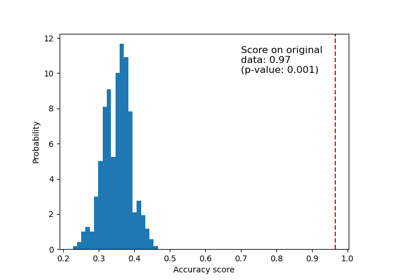# sklearn.model_selection.permutation_test_score¶

sklearn.model_selection.permutation_test_score(estimator, X, y, *, groups=None, cv=None, n_permutations=100, n_jobs=None, random_state=0, verbose=0, scoring=None, fit_params=None)[source]

Evaluate the significance of a cross-validated score with permutations.

Permutes targets to generate ‘randomized data’ and compute the empirical p-value against the null hypothesis that features and targets are independent.

The p-value represents the fraction of randomized data sets where the estimator performed as well or better than in the original data. A small p-value suggests that there is a real dependency between features and targets which has been used by the estimator to give good predictions. A large p-value may be due to lack of real dependency between features and targets or the estimator was not able to use the dependency to give good predictions.

Read more in the User Guide.

Parameters:
estimatorestimator object implementing ‘fit’

The object to use to fit the data.

Xarray-like of shape at least 2D

The data to fit.

yarray-like of shape (n_samples,) or (n_samples, n_outputs) or None

The target variable to try to predict in the case of supervised learning.

groupsarray-like of shape (n_samples,), default=None

Labels to constrain permutation within groups, i.e. y values are permuted among samples with the same group identifier. When not specified, y values are permuted among all samples.

When a grouped cross-validator is used, the group labels are also passed on to the split method of the cross-validator. The cross-validator uses them for grouping the samples while splitting the dataset into train/test set.

cvint, cross-validation generator or an iterable, default=None

Determines the cross-validation splitting strategy. Possible inputs for cv are:

• None, to use the default 5-fold cross validation,

• int, to specify the number of folds in a (Stratified)KFold,

• An iterable yielding (train, test) splits as arrays of indices.

For int/None inputs, if the estimator is a classifier and y is either binary or multiclass, StratifiedKFold is used. In all other cases, KFold is used. These splitters are instantiated with shuffle=False so the splits will be the same across calls.

Refer User Guide for the various cross-validation strategies that can be used here.

Changed in version 0.22: cv default value if None changed from 3-fold to 5-fold.

n_permutationsint, default=100

Number of times to permute y.

n_jobsint, default=None

Number of jobs to run in parallel. Training the estimator and computing the cross-validated score are parallelized over the permutations. None means 1 unless in a joblib.parallel_backend context. -1 means using all processors. See Glossary for more details.

random_stateint, RandomState instance or None, default=0

Pass an int for reproducible output for permutation of y values among samples. See Glossary.

verboseint, default=0

The verbosity level.

scoringstr or callable, default=None

A single str (see The scoring parameter: defining model evaluation rules) or a callable (see Defining your scoring strategy from metric functions) to evaluate the predictions on the test set.

If None the estimator’s score method is used.

fit_paramsdict, default=None

Parameters to pass to the fit method of the estimator.

New in version 0.24.

Returns:
scorefloat

The true score without permuting targets.

permutation_scoresarray of shape (n_permutations,)

The scores obtained for each permutations.

pvaluefloat

The p-value, which approximates the probability that the score would be obtained by chance. This is calculated as:

(C + 1) / (n_permutations + 1)

Where C is the number of permutations whose score >= the true score.

The best possible p-value is 1/(n_permutations + 1), the worst is 1.0.

Notes

This function implements Test 1 in:

Ojala and Garriga. Permutation Tests for Studying Classifier Performance. The Journal of Machine Learning Research (2010) vol. 11

## Examples using sklearn.model_selection.permutation_test_score¶Test with permutations the significance of a classification score

Test with permutations the significance of a classification score